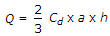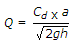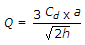# Mechanical Engineering - Hydraulics and Fluid Mechanics

### Exercise :: Hydraulics and Fluid Mechanics - Section 3

41.

The discharge through a small rectangular orifice is given by (where Cd = Coefficient of discharge for the orifice, a = Cross-sectional area of the orifice, h = Height of the liquid above the centre of the orifice)

 A. Q = Cd x a x 2gh B.C.D.Answer: Option A

Explanation:

No answer description available for this question. Let us discuss.

42.

The siphon will work satisfactorily, if the minimum pressure in the pipe is __________ vapour pressure of liquid.

 A. equal to B. less than C. more than

Answer: Option C

Explanation:

No answer description available for this question. Let us discuss.

43.

The difference between the notch and weir is that the notch is of bigger size and the weir is of a smaller size.

 A. Agree B. Disagree

Answer: Option B

Explanation:

No answer description available for this question. Let us discuss.

44.

An open tank containing liquid is moving with an acceleration on an inclined plane. The inclination of the free surface of the liquid will be __________ to the acceleration of the tank.

 A. equal to B. directly proportional C. inversely proportional

Answer: Option B

Explanation:

No answer description available for this question. Let us discuss.

45.

Euler's number is the ratio of __________ force to pressure force.

 A. inertia B. gravity C. viscous

Answer: Option A

Explanation:

No answer description available for this question. Let us discuss.

#### Current Affairs 2021

Interview Questions and Answers